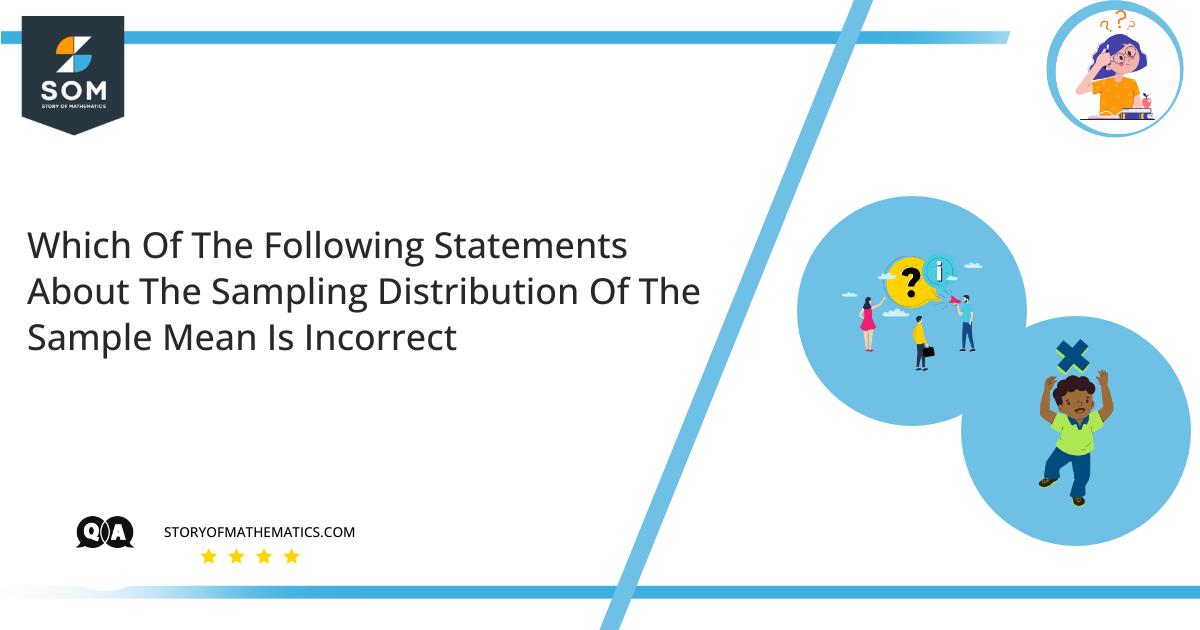# Which of the following statements about the sampling distribution of the sample mean is incorrect?•  The standard deviation of the sampling distribution will decrease as the sample size increases.
• The standard deviation of a sampling distribution is a measure of the variability of the sample mean among repeated samples.
• The sample mean is an unbiased estimate of the population mean.
• The sampling distribution shows how the sample means will vary in repeated samples.
• The sampling distribution depicts how the sample was distributed around the sample mean.

The main objective of this question is to choose the incorrect statement about the sampling distribution of sample mean out of the given five statements.

Theoretically, the sampling distribution of a data set is the probability distribution of that data set. A sampling distribution is a relative frequency distribution with an extremely large number of samples. More accurately, as the number of samples tends to reaches infinity, a relative frequency distribution tends to the sampling distribution.

Similarly, we can collect a large number of individual outcomes and combine them to construct a distribution with a center and spread. If we take a large number of samples having the same size, and compute the mean of each of them, we can combine those means to construct a distribution. This new distribution is then said to be the sampling distribution of sample means.

• True, because a larger sample provides so much information about the population which enables more accurate predictions. If the predictions are more accurate, the variability (as estimated by the standard deviation) is also reduced.
• True, since the variability of the sample means over all possible samples is represented by the standard deviation of the sampling distribution of the sample mean.
• True, the sample mean is an unbiased estimator of the population mean.
• True, since the variation is provided by the standard deviation of the sampling distribution.
• False, Because the sampling distribution is the distribution of all possible sample means, it cannot be centered around the sample mean since there are many sample means.

Hence, “The sampling distribution shows how the sample was distributed around the sample mean” is incorrect.

## Example

A rowing team is made up of four rowers weighing $100, 56, 146$, and $211$ pounds. Determine the sample mean for each of the possible random samples with replacement of size two. Also, calculate the probability distribution, the mean, and standard deviation of the sample mean $\bar{x}$.

### Numerical Solution

The table below shows all possible samples with size two replacement, as well as the mean of each sample:

 Sample Mean Sample Mean Sample Mean Sample Mean $100,100$ $100$ $56,100$ $78$ $146,100$ $123$ $211,100$ $155.5$ $100,56$ $78$ $56,56$ $56$ $146,56$ $101$ $211,56$ $133.5$ $100,146$ $123$ $56,146$ $101$ $146,146$ $146$ $211,146$ $178.5$ $100,211$ $155.5$ $56,211$ $133.5$ $146,211$ $178.5$ $211,211$ $211$

Because the $16$ samples are all equally likely, we can simply count to obtain the probability distribution of the sample mean:

 $\bar{x}$ $56$ $78$ $100$ $101$ $123$ $133.5$ $146$ $155.5$ $178.5$ $211$ $P(\bar{x})$ $\dfrac{1}{16}$ $\dfrac{2}{16}$ $\dfrac{1}{16}$ $\dfrac{2}{16}$ $\dfrac{2}{16}$ $\dfrac{2}{16}$ $\dfrac{1}{16}$ $\dfrac{2}{16}$ $\dfrac{2}{16}$ $\dfrac{1}{16}$

$\mu_{\bar{x}}=\sum\bar{x}P(\bar{x})$

$=56\left(\dfrac{1}{16}\right)+ 78\left(\dfrac{2}{16}\right)+ 100\left(\dfrac{1}{16}\right)+ 101\left(\dfrac{2}{16}\right)+ 123\left(\dfrac{2}{16}\right)+$

$133.5\left(\dfrac{2}{16}\right)+ 146\left(\dfrac{1}{16}\right)+ 155.5\left(\dfrac{2}{16}\right)+ 178.5\left(\dfrac{2}{16}\right)+ 211\left(\dfrac{1}{16}\right)=128.25$

Now, compute:

$\sum\bar{x}^2P(\bar{x})=(56)^2\left(\dfrac{1}{16}\right)+ (78)^2\left(\dfrac{2}{16}\right)+ (100)^2\left(\dfrac{1}{16}\right)+ (101)^2\left(\dfrac{2}{16}\right)$

$+ (123)^2\left(\dfrac{2}{16}\right)+ (133.5)^2\left(\dfrac{2}{16}\right)+ (146)^2\left(\dfrac{1}{16}\right)$

$+ (155.5)^2\left(\dfrac{2}{16}\right)+ (178.5)^2\left(\dfrac{2}{16}\right)+ (211)^2\left(\dfrac{1}{16}\right)=18095.65625$

So,  $\sigma_{\bar{x}}=\sqrt{\sum\bar{x}^2P(\bar{x})-(\sum\bar{x}P(\bar{x}))^2}$

$=\sqrt{18095.65625-(128.25)^2}=40.59$